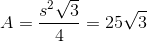## Example Questions

### Example Question #2 : How To Find The Area Of An Equilateral Triangle

The area of square ABCD is 50% greater than the perimeter of the equilateral triangle EFG. If the area of square ABCD is equal to 45, then what is the area of EFG?

50√3

25√3

30

25

50

25√3

Explanation:

If the area of ABCD is equal to 45, then the perimeter of EFG is equal to x * 1.5 = 45. 45 / 1.5 = 30, so the perimeter of EFG is equal to 30. This means that each side is equal to 10.

The height of the equilateral triangle EFG creates two 30-60-90 triangles, each with a hypotenuse of 10 and a short side equal to 5. We know that the long side of 30-60-90 triangle (here the height of EFG) is equal to √3 times the short side, or 5√3.

We then apply the formula for the area of a triangle, which is 1/2 * b * h. We get 1/2 * 10 * 5√3 = 5 * 5√3 = 25√3.

In general, the height of an equilateral triangle is equal to √3 / 2 times a side of the equilateral triangle. The area of an equilateral triangle is equal to 1/2 * √3s/ 2 * s = √3s2/4.

### Example Question #3 : How To Find The Area Of An Equilateral Triangle

What is the area of an equilateral triangle with sides 12 cm?

72√3

12√2

54√2

36√3

18√3

36√3

Explanation:

An equilateral triangle has three congruent sides and results in three congruent angles. This figure results in two special right triangles back to back: 30° – 60° – 90° giving sides of x - x √3 – 2x in general. The height of the triangle is the x √3 side.  So Atriangle = 1/2 bh = 1/2 * 12 * 6√3 = 36√3 cm2.

### Example Question #1 : Equilateral Triangles

An equilateral triangle has a perimeter of 18. What is its area?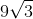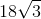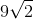Explanation:

Recall that an equilateral triangle also obeys the rules of isosceles triangles.   That means that our triangle can be represented as having a height that bisects both the opposite side and the angle from which the height is "dropped."  For our triangle, this can be represented as: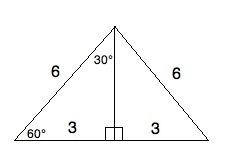Now, although we do not yet know the height, we do know from our 30-60-90 regular triangle that the side opposite the 60° angle is √3 times the length of the side across from the 30° angle. Therefore, we know that the height is 3√3.

Now, the area of a triangle is (1/2)bh. If the height is 3√3 and the base is 6, then the area is (1/2) * 6 * 3√3 = 3 * 3√3 = 9√(3).

### Example Question #1 : Equilateral Triangles

A regular hexagon and an equilateral triangle have equal perimeters.  If the hexagon has side lengths of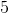, what is the area of the triangle?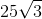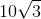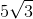Perimeter of hexagon =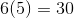= perimeter of triangle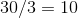= side of the triangle